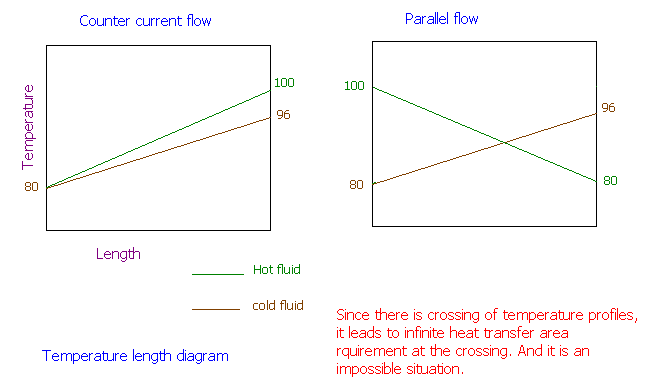### Heat Exchanger Flow Arrangement

A heat exchanger heats 25,000 kg/hr of water entering at 80oC while cooling 20,000 kg/hr of water from 100oC to 80oC. Determine the heat transfer area necessary for (i) Parallel flow arrangement (ii) Counter flow arrangement. Given Overall heat transfer coefficient, U= 1,500 W/m2.

Calculations:

Heat of heat transferred by the cooling water = Q = mCpΔT = 20000 x 4.184 x (100 - 80) = 1673600 kJ/hr

This will be the amount of heat transferred to the water that is getting heated up. Therefore, temperature change is = 1673600 / (25000 x 4.184) = 16oC

i.e., outlet temperature = 80 + 16 = 96oC